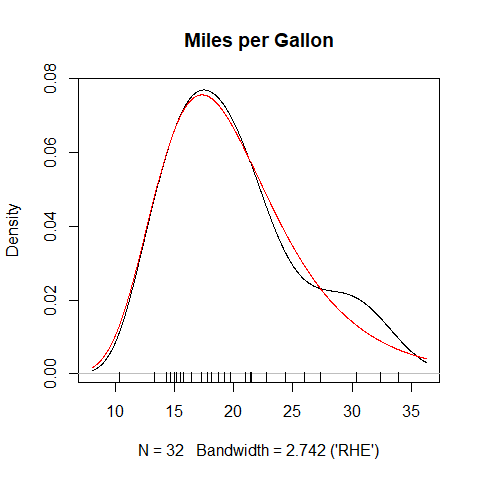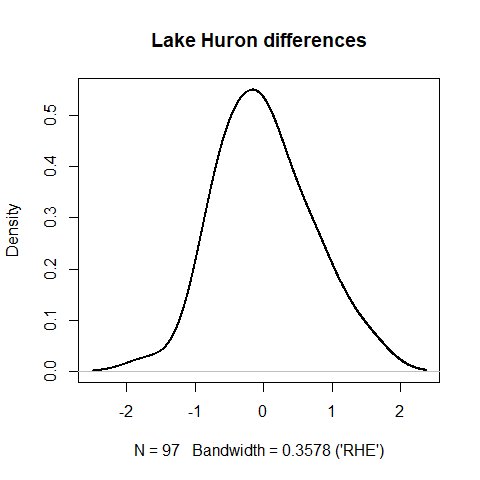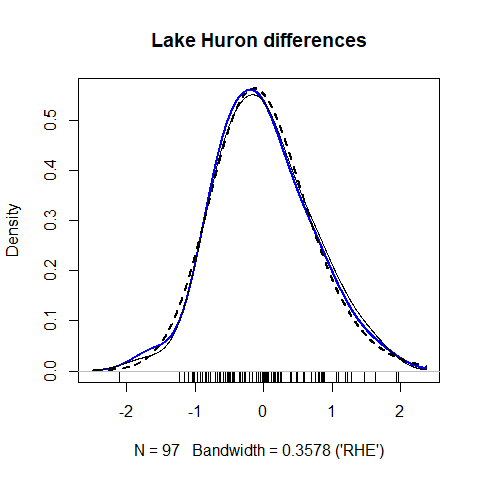Basic usage

kdensity is called using a syntax similar to stats::density, but with some additional arguments. A call to kdensity returns a density function (with class kdensity), which can used as ordinary R-function:

library("kdensity")
kde = kdensity(mtcars$mpg, start = "gumbel", kernel = "gaussian") kde(20) #>  0.06829002 Hence it can be used to calculate functionals, such as the expectation: integrate(function(x) x*kde(x), lower = -Inf, upper = Inf)$value
#>  20.15896

Plotting works as with stats::density. If kdensity was called with a parametric start, use plot_start = TRUE inside a plotting function in order to plot the estimated parametric start density instead of the kernel density.

plot(kde, main = "Miles per Gallon")
lines(kde, plot_start = TRUE, col = "red")
rug(mtcars$mpg)If the R-package magrittr is installed, you can use pipes to plot: library("magrittr") kde %>% plot(main = "Miles per Gallon") %>% lines(plot_start = TRUE, col = "red") The generics coef, logLik, AIC and BIC are supported, but work only on the parametric start. coef(kde) #> loc scale #> 17.321183 4.864584 logLik(kde) #> 'log Lik.' 1.644676 (df=2) AIC(kde) #>  0.710647 To view information about the kernel density, use the summary generic. summary(kde) #> #> Call: #> kdensity(x = mtcars$mpg, kernel = "gaussian", start = "gumbel")
#>
#> Data:        mtcars$mpg (32 obs.) #> Bandwidth: 2.742 ('RHE') #> Support: (-Inf, Inf) #> Kernel: gaussian #> Start: gumbel #> Range: (10.4, 33.9) #> NAs in data: FALSE #> Adjustment: 1 #> #> Parameter estimates: #> loc: 17.32 #> scale: 4.865 Access members of the kernel density with$ or [[.

kde$start_str #>  "gumbel" kde[["x"]] #>  21.0 21.0 22.8 21.4 18.7 18.1 14.3 24.4 22.8 19.2 17.8 16.4 17.3 15.2 #>  10.4 10.4 14.7 32.4 30.4 33.9 21.5 15.5 15.2 13.3 19.2 27.3 26.0 30.4 #>  15.8 19.7 15.0 21.4 To make changes to the kernel density estimator, you can use the generic update function, replace values with$, or make a new one.

kde\$bw = "RHE"
update(kde, start = "normal")

Custom densities and kernels

Parametric starts

You must supply two functions and one vector to kdensity in order to use a custom parametric start. The first is a density function, the second is a function that estimates the named parameters of the density, and the third is the support of the density.

The density function must take the data as its first argument, and all its parameters must be named. In addition, the function estimator must return a vector containing named parameters that partially match the parameter names of the density function. For instance, the arguments of dnorm are x, mean, sd, log, where log = TRUE means that the logarithm of the density is returned. Since estimator returns a named vector with names mean and sd, the names are completely matched.

The estimator function doesn’t need to be simple, as the next example shows.

Example: A skew hyperbolic t-distribution start

The built-in data set LakeHuron contains annual measurements of the water level of Lake Huron from 1875 to 1972, measured in feet. We will take a look at the distribution of differences in water level across two consecutive years. Since the data are supported on the real line and there is no good reason to assume anything else, we will use a normal start.The density is clearly non-normal. Still, it looks fairly regular, and it should be possible to model it parametrically with some success. One of many parametric families of distributions more flexible than the normal family is the skew hyperbolic t-distribution, which is implemented in the R package SkewHyperbolic. This package contains the density function dskewhyp and a maximum likelihood estimating function in skewhypFit. Using these functions, we make the following list:

Now we are ready to run kdensity and do some plotting. Note the plot option plot_start = TRUE. With this option on, the estimated parametric density is plotted without any non-parametric correction.Since all the curves are in agreement, we are justified in using the skew hyperbolic t-distribution for further analysis.

Kernels

If you want to make custom kernel, you will need to supply the kernel function, with arguments y, x, h. Here x is the random data you put into kdensity, h is the final bandwidth, and y is the point you want to evaluate at. The kernel is called as 1/h*kernel(y, x, h), and should be vectorized in both x and y. In addition to the kernel function, you must supply a support argument, which states the domain of definition of the kernel. For instance, the gcopula kernel is defined on c(0, 1). In addition, you can optionally supply the standard deviation of the kernel. This is only used for symmetric kernels, and is useful since it makes them comparable. For example, the implementation of the gaussian kernel is

Custom kernels can be complicated, and do not have to be symmetric.